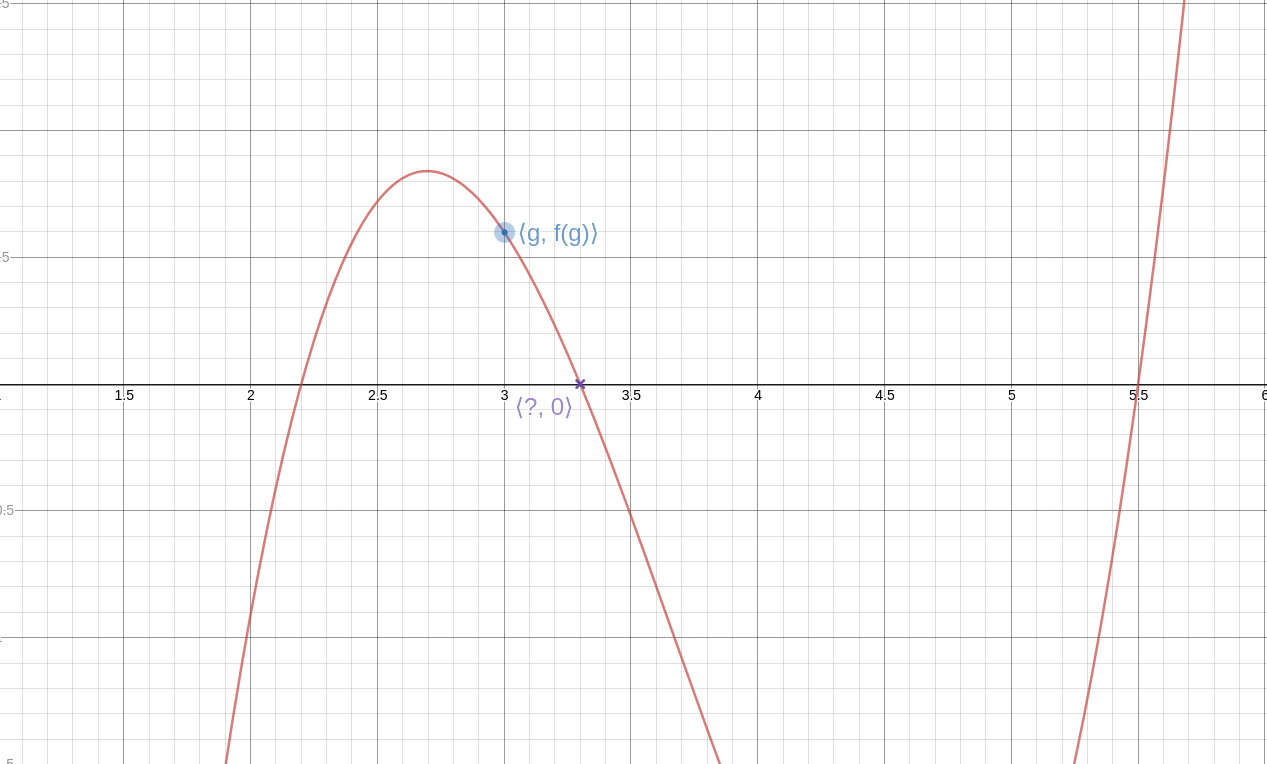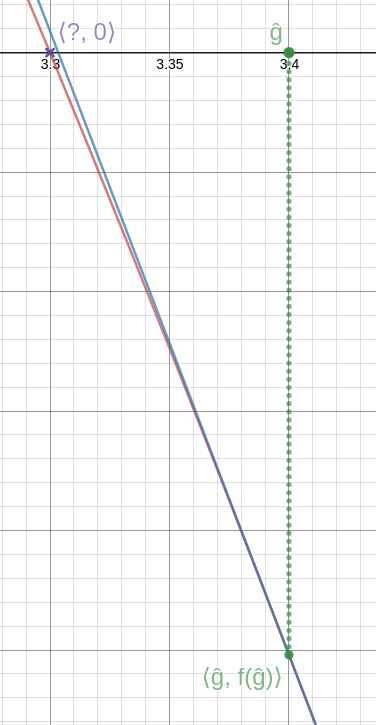# The Universe of Discourse

Tue, 13 Oct 2020

Newton's method goes like this: We have a function !!f!! and we want to solve the equation !!f(x) = 0.!! We guess an approximate solution, !!g!!, and it doesn't have to be a very good guess.Then we calculate the line !!T!! tangent to !!f!! through the point !!\langle g, f(g)\rangle!!. This line intersects the !!x!!-axis at some new point !!\langle \hat g, 0\rangle!!, and this new value, !!\hat g!!, is a better approximation to the value we're seeking.Analytically, we have:

$$\hat g = g - \frac{f(g)}{f'(g)}$$

where !!f'(g)!! is the derivative of !!f!! at !!g!!.

We can repeat the process if we like, getting better and better approximations to the solution. (See detail at left; click to enlarge. Again, the blue line is the tangent, this time at !!\langle \hat g, f(\hat g)\rangle!!. As you can see, it intersects the axis very close to the actual solution.)

In general, this requires calculus or something like it, but in any particular case you can avoid the calculus. Suppose we would like to find the square root of 2. This amounts to solving the equation $$x^2-2 = 0.$$ The function !!f!! here is !!x^2-2!!, and !!f'!! is !!2x!!. Once we know (or guess) !!f'!!, no further calculus is needed. The method then becomes: Guess !!g!!, then calculate $$\hat g = g - \frac{g^2-2}{2g}.$$ For example, if our initial guess is !!g = 1.5!!, then the formula above tells us that a better guess is !!\hat g = 1.5 - \frac{2.25 - 2}{3} = 1.4166\ldots!!, and repeating the process with !!\hat g!! produces !!1.41421\mathbf{5686}!!, which is very close to the correct result !!1.41421\mathbf{3562}!!. If we want the square root of a different number !!n!! we just substitute it for the !!2!! in the numerator.

This method for extracting square roots works well and requires no calculus. It's called the Babylonian method and while there's no evidence that it was actually known to the Babylonians, it is quite ancient; it was first recorded by Hero of Alexandria about 2000 years ago.

How might this have been discovered if you didn't have calculus? It's actually quite easy. Here's a picture of the number line. Zero is at one end, !!n!! is at the other, and somewhere in between is !!\sqrt n!!, which we want to find.Also somewhere in between is our guess !!g!!. Say we guessed too low, so !!0 \lt g < \sqrt n!!. Now consider !!\frac ng!!. Since !!g!! is too small to be !!\sqrt n!! exactly, !!\frac ng!! must be too large. (If !!g!! and !!\frac ng!! were both smaller than !!\sqrt n!!, then their product would be smaller than !!n!!, and it isn't.)Similarly, if the guess !!g!! is too large, so that !!\sqrt n < g!!, then !!\frac ng!! must be less than !!\sqrt n!!. The important point is that !!\sqrt n!! is between !!g!! and !!\frac ng!!. We have narrowed down the interval in which !!\sqrt n!! lies, just by guessing.

Since !!\sqrt n!! lies in the interval between !!g!! and !!\frac ng!! our next guess should be somewhere in this smaller interval. The most obvious thing we can do is to pick the point halfway in the middle of !!g!! and !!\frac ng!!, So if we guess the average, $$\frac12\left(g + \frac ng\right),$$ this will probably be much closer to !!\sqrt n!! than !!g!! was:This average is exactly what Newton's method would have calculated, because $$\frac12\left(g + \frac ng\right) = g - \frac{g^2-n}{2g}.$$

But we were able to arrive at the same computation with no calculus at all — which is why this method could have been, and was, discovered 1700 years before Newton's method itself.

If we're dealing with rational numbers then we might write !!g=\frac ab!!, and then instead of replacing our guess !!g!! with a better guess !!\frac12\left(g + \frac ng\right)!!, we could think of it as replacing our guess !!\frac ab!! with a better guess !!\frac12\left(\frac ab + \frac n{\frac ab}\right)!!. This simplifies to

$$\frac ab \Rightarrow \frac{a^2 + nb^2}{2ab}$$

so that for example, if we are calculating !!\sqrt 2!!, and we start with the guess !!g=\frac32!!, the next guess is $$\frac{3^2 + 2\cdot2^2}{2\cdot3\cdot 2} = \frac{17}{12} = 1.4166\ldots$$ as we saw before. The approximation after that is !!\frac{289+288}{2\cdot17\cdot12} = \frac{577}{408} = 1.41421568\ldots!!. Used this way, the method requires only integer calculations, and converges very quickly.

But the numerators and denominators increase rapidly, which is good in one sense (it means you get to the accurate approximations quickly) but can also be troublesome because the numbers get big and also because you have to multiply, and multiplication is hard.

But remember how we figured out to do this calculation in the first place: all we're really trying to do is find a number in between !!g!! and !!\frac ng!!. We did that the first way that came to mind, by averaging. But perhaps there's a simpler operation that we could use instead, something even easier to compute?

Indeed there is! We can calculate the mediant. The mediant of !!\frac ab!! and !!\frac cd!! is simply $$\frac{a+c}{b+d}$$ and it is very easy to show that it lies between !!\frac ab!! and !!\frac cd!!, as we want.

So instead of the relatively complicated $$\frac ab \Rightarrow \frac{a^2 + nb^2}{2ab}$$ operation, we can try the very simple and quick $$\frac ab \Rightarrow \operatorname{mediant}\left(\frac ab, \frac{nb}{a}\right) = \frac{a+nb}{b+a}$$ operation.

Taking !!n=2!! as before, and starting with !!\frac 32!!, this produces:

$$\frac 32 \Rightarrow\frac{ 7 }{ 5 } \Rightarrow\frac{ 17 }{ 12 } \Rightarrow\frac{ 41 }{ 29 } \Rightarrow\frac{ 99 }{ 70 } \Rightarrow\frac{ 239 }{ 169 } \Rightarrow\frac{ 577 }{ 408 } \Rightarrow\cdots$$

which you may recognize as the convergents of !!\sqrt2!!. These are actually the rational approximations of !!\sqrt 2!! that are optimally accurate relative to the sizes of their denominators. Notice that !!\frac{17}{12}!! and !!\frac{577}{408}!! are in there as they were before, although it takes longer to get to them.

I think it's cool that you can view it as a highly-simplified version of Newton's method.

[ Addendum: An earlier version of the last paragraph claimed:

None of this is a big surprise, because it's well-known that you can get the convergents of !!\sqrt n!! by applying the transformation !!\frac ab\Rightarrow \frac{a+nb}{a+b}!!, starting with !!\frac11!!.

Simon Tatham pointed out that this was mistaken. It's true when !!n=2!!, but not in general. The sequence of fractions that you get does indeed converge to !!\sqrt n!!, but it's not usually the convergents, or even in lowest terms. When !!n=3!!, for example, the numerators and denominators are all even. ]

[ Addendum: Newton's method as I described it, with the crucial !!g → g - \frac{f(g)}{f'(g)}!! transformation, was actually invented in 1740 by Thomas Simpson. Both Isaac Newton and Thomas Raphson had earlier described only special cases, as had several Asian mathematicians, including Seki Kōwa. ]

[ Previous discussion of convergents: Archimedes and the square root of 3; 60-degree angles on a lattice. A different variation on the Babylonian method. ]

[ Note to self: Take a look at what the AM-GM inequality has to say about the behavior of !!\hat g!!. ]

[ Addendum 20201018: A while back I discussed the general method of picking a function !!f!! that has !!\sqrt 2!! as a fixed point, and iterating !!f!!. This is yet another example of such a function. ]